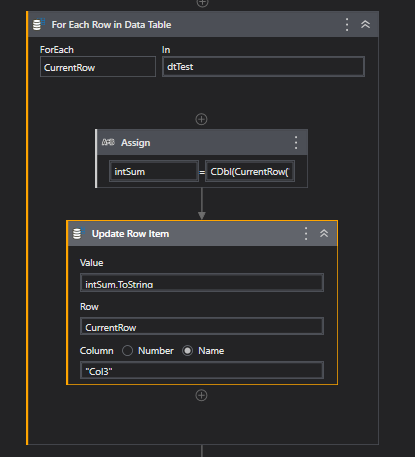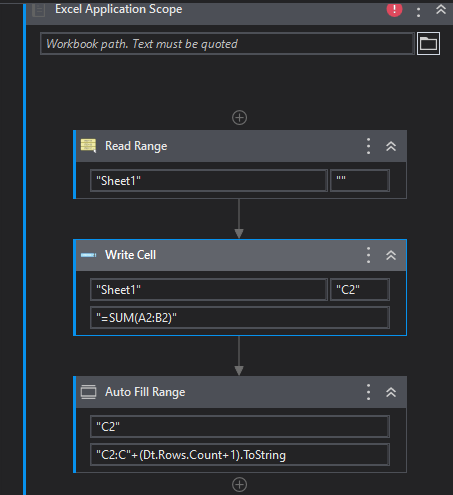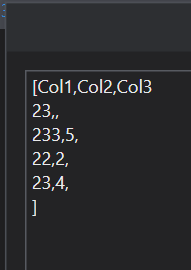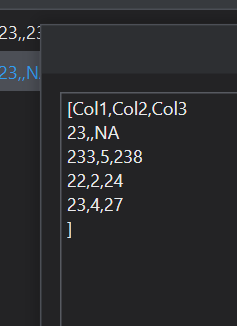# Dear Team, How to sum col1 and col2 data and put result in col3 with out using for each in excel

How to sum col1 and col2 data and put the result in col3 with out using each in excel.

Hi @Lokesh_M2 ,

Sum Col1 and Col2, then update Col3

intSum =CDbl(CurrentRow(“Col1”))+CDbl(CurrentRow(“Col2”))Thanks,
Rajkumar

Thank u. But i need with out using FOR EACH

Try thisRegards
Sudharsan

Dt is the variable from the read range @Lokesh_M2

Hi @Lokesh_M2 ,

add Column as “Col3” in dtTest, then use below expression

``````> (From SV In dtTest.AsEnumerable()
> Let Col3= If(IsNumeric(SV("Col1").ToString) And IsNumeric(SV("Col2").ToString),CStr(CDbl(SV("Col1").ToString)+CDbl(SV("Col2").ToString)),"NA")
``````

Excel.xaml (7.5 KB)

InputOutputthanks
Rajkumar

option strict on disallows implicit conversions from ‘string’ to ‘long’
Showing above error